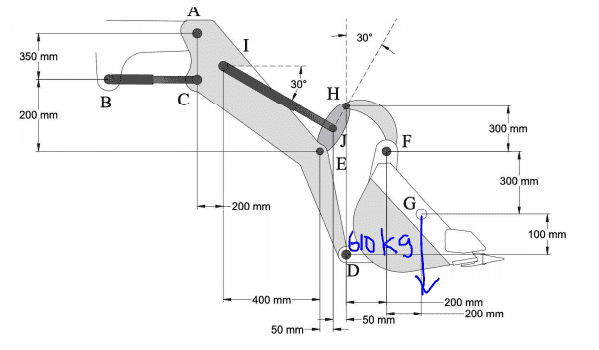# Machine Analysis: Statics

• Engineering
sam0916
Homework Statement:
A scoop carries 610 kg of load with center of mass at G. Neglecting the mass of the
members, calculate the hydraulic force in IJ, (Use g = 9.81 m/s2). Fij = = 11, 051.9516 N (T).
Relevant Equations:
A scoop carries 610 kg of load with center of mass at G. Neglecting the mass of the
members, calculate the hydraulic force in IJ, (Use g = 9.81 m/s2). Fij = = 11, 051.9516 N (T).Do you guys have techniques in solving for the Force in hydraulic IJ? I've been stucked for a while now and I can't seem to find the answer.The question says the answer should be = 11, 051.9516 N (T).